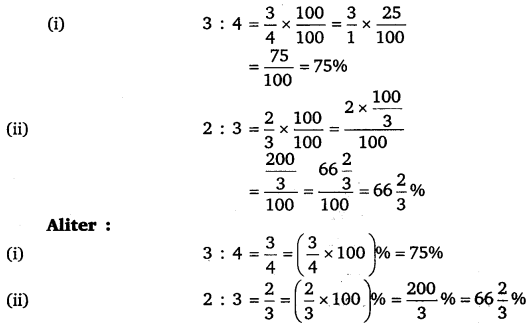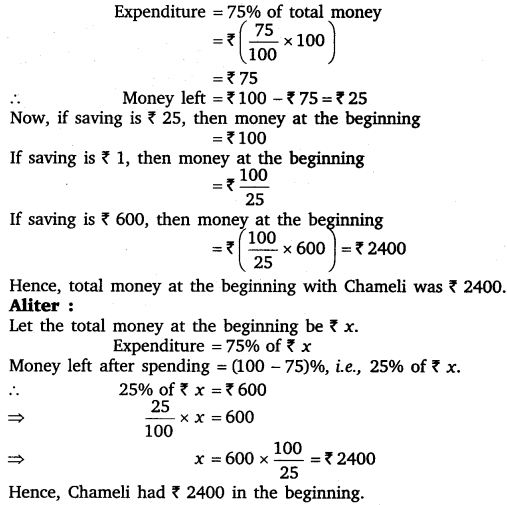# Class 8 Maths NCERT Solutions for Chapter – 8 Comparing Quantities Ex – 8.1

## Comparing Quantities

Question 1.
Find the ratio of the following :
(a)
Speed of a cycle is 15 km per hour to the speed of scooter 30 km per hour.
(b) 5 m to 10 km
(c) 50 paise to ₹ 5

Solution:
(a) Speed of cycle which is 15 km/hr to the speed of scooter which is 30 km/hr = 15 km : 30 km = 1:2.

(b) 5 m to 10 km = 5m : 10 km [∵ 1 km = 1000 m, ∴ 10 km = 10000 m]
= 5 m : 10000 m
= 1 : 2000
[Dividing the 1st and 2nd terms by their HCF = 5]

(c) 50 paise to ₹ 5 = 50 paise : ₹ 5 [₹ 1 = 100 paise, ₹ 5 = 500 paise]
= 50 paise : 500 paise
= 1 : 10
[Dividing the 1st and 2nd terms by their HCF = 50]

Question 2.
Convert the following ratios to percentages :
(a)
3 : 4
(b) 2 : 3

Solution:Question 3.
72% of 25 students are good in mathematics. How many are not good in mathematics?

Solution:
72% of 25 students are good in mathematics.
∴ (100 – 72)%, i. e., 28% of 25 students are not good in mathematics.
Thus, their number = 28% of 25$=\left( \frac { 28 }{ 100 } \times 25 \right) =7$ students

Question 4.
A football team won 10 matches out of the total number of matches they played. If their win percentage was 40, then how many matches did they play in all?

Solution:
Let x games be played in all.
Since 40% of the total games is given as 10,
∴ 40% of x = 10
⇒$\frac { 40 }{ 100 } \times x=10$
⇒$x=\frac { 100 }{ 40 } \times 10=25$

Question 5.
If Chameli had ₹ 600 left after spending 75% of her money, how much did she have in the beginning?

Solution:
Suppose total money with Chameli at the beginning be ₹ 100.Question 6.
If 60% people in a city like cricket, 30% like football and the remaining like other games, then what per cent of the people like the other games? If the total number of people are 50 lakh, find the exact number who like each type of game.

Solution:
We have,
People who like cricket = 60%
People who like football = 30%
∴ People who like other games = (100 – 60 – 30)% =10%
Total number of people = 5000000
∴ People who like cricket =$\frac { 60 }{ 100 } \times 5000000$
= 3000000 = 30 lakh
People who like football =$\frac { 30 }{ 100 } \times 5000000$
= 1500000 =15 lakh
and, the people who like other games$\frac { 10 }{ 100 } \times 5000000$
= 500000 = 5 lakh.

### Take Your IIT JEE Coaching to Next Level with Examtube

• Mentoring & Teaching by IITians
• Regular Testing & Analysis
• Preparation for Various Engineering Entrance Exams
• Support for School/Board Exams